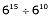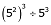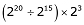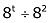# Class 7 Maths Chapter 13 Exponents and Powers

### Exercise-13.1

Question 1:

Find the value of:

(i) 26

(ii) 93

(iii) 112

(iv) 54

(i)In the given question,

We have to find the value of 26

We have,

26 = 2 × 2 × 2 × 2 × 2 × 2= 64

(ii) In the given question,

We have to find the value of 93

We have,

93 = 9 × 9 × 9 = 729

(iii) In the given question,

We have to find the value of 112

We have,

112 = 11 × 11 = 121

(iv) In the given question,

We have to find the value of 54

We have,

54 = 5 × 5 × 5 × 5= 625

Question 2:

Express the following in exponential form:

(i) 6 × 6 × 6 × 6

(ii) t × t

(iii) b × b × b × b

(iv) 5 × 5 × 7 × 7 × 7

(v) 2 × 2 × a × a

(vi) a × a × a × c × c × c × d

(i) In the given question,

We have to express the given expression into exponential form

We have,

6 × 6 × 6 × 6 = 64

(ii) In the given question,

We have to express the given expression into exponential form

We have,

t × t = t2

(iii) In the given question,

We have to express the given expression into exponential form

We have,

b × b × b × b = b4

(iv) In the given question,

We have to express the given expression into exponential form

We have,

5 × 5 × 7 × 7 × 7 = 52 × 73

(v) In the given question,

We have to express the given expression into exponential form

We have,

2 × 2 × a × a = 22 × a2

(vi) In the given question,

We have to express the given expression into exponential form

We have,

a × a × a × c × c × c × c × d = a3 × c4 × d

Question 3:

Express each of the following numbers using the exponential notation:

(i) 512

(ii) 343

(iii) 729

(iv) 3125

(i) In the given question,

We have to express the given numbers into exponential notation

We have,

512 = 2 × 2 × 2 × 2 × 2 × 2 × 2 × 2 × 2 = 29

(ii) In the given question,

We have to express the given numbers into exponential notation

We have,

343 = 7 × 7 × 7 = 73

(iii) In the given question,

We have to express the given numbers into exponential notation

We have,

729 = 3 × 3 × 3 × 3 × 3 × 3 = 36

(iv) In the given question,

We have to express the given numbers into exponential notation

We have,

3125 = 5 × 5 × 5 × 5 × 5 = 55

Question 4:

Identify the greater number, wherever possible, in each of the following.

### Exercise-13.2

Question 1:

Using laws of exponents simplify and write the answer in exponential form:

(i) 32 × 34 × 38

(ii)(iii)  a3  × a2

(iv) 7x × 72

(v)(vi)25 × 52

(vii)a4 × b4

(viii)(34)3

(ix)(x)(i) We have,

32× 34 × 38

We know that,

(am× an = am + n)

Thus,

32× 34 × 38

= (3)2 + 4 + 8

= 314

(ii) We have,

615 610

We know that,

(am an = am – n)

Thus,

615 610

= (6)15 – 10

= 65

(iii) We have,

a3× a2

We know that,

(am× an = am + n)

Therefore,

a3× a2

= (a)3 + 2

= a5

(iv) We have,

7x × 72

We know that,

(am × an = am + n)

Thus,

7x × 72

= (7)x + 2

(v) We have,

(52)3 53

Using identity:

(am)n= am × n

= 52 × 3 53

= 56 53

We know that,

(am an = am – n)

Thus,

56 53

= (5)6 – 3

= 53

(vi) We have,

25× 55

We know that,

[am ×bm = (a × b)m]

Thus,

25× 55

= (2 × 5)5 + 5

= 105

(vii) We have,

a4 × b4

We know that,

[am × bm = (a × b)m]

Thus,

a4 × b4

= (a × b)4

(viii) We have,

(34)3

We know that,

(am)n = amn)

Thus,

(34)3

= (34)3

= 312

(ix) We have,

(220 215) × 23

We know that,

(am an = am – n)

Thus,

(220 – 15) × 23

= (2)× 23

We know that,

(am × an = am + n)

Thus,

(2)× 23

=(25 + 3)

= 28

(x) We have,

(8t 82)

We know that,

(am an = am – n)

Thus,

(8t 82)

= (8t – 2)

### Exercise-13.3

Get 30% off your first purchase!

X
error: Content is protected !!
Scroll to Top